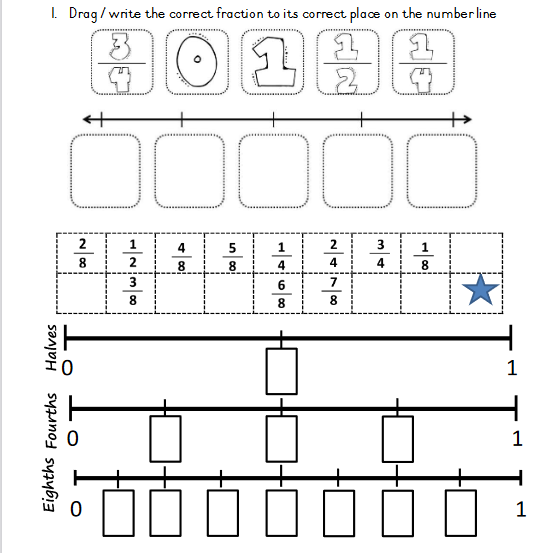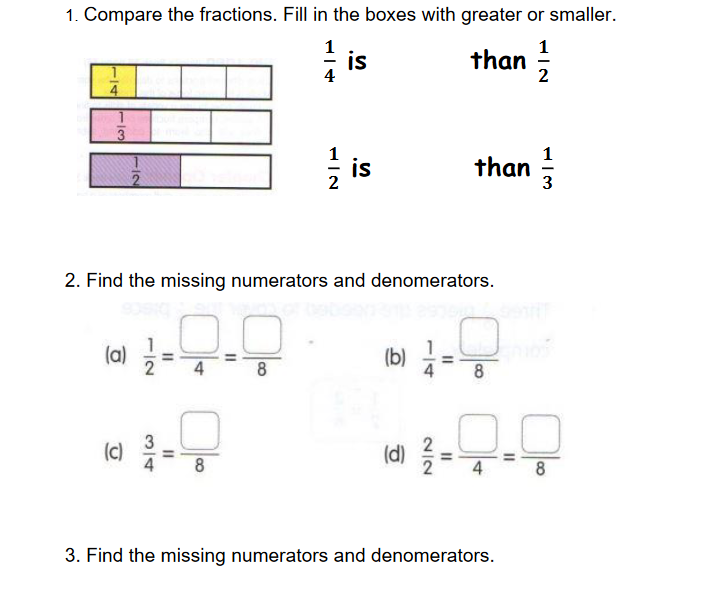# Lesson Notes By Weeks and Term - Primary 3

Fractions

TERM: 3rd Term

WEEK: 4

CLASS: Primary 3

AGE: 8 years

DURATION: 5 periods of 40 minutes each

DATE:

SUBJECT: Mathematics

TOPIC: Fractions

SPECIFIC OBJECTIVES: At the end of the lesson, the pupils should be able to

1. Solve word problems in context and explain own solutions to problems that involve equal sharing leading to solutions that include unitary and non-unitary fractions, e.g. ½ â ¼ ¹/5
2. Use and name fractions including halves, quarters, thirds and fifths.
3. Recognise fractions in diagrammatic form.
4. Write fractions as 1 half, 2 thirds.

INSTRUCTIONAL TECHNIQUES: Explanation, question and answer, demonstration, practical, assessments

INSTRUCTIONAL MATERIALS: Fraction squares, fraction circles, Whiteboards/scrap paper, Fraction strips, videos from source-

PERIOD 1: Sharing leading to fractions

 PRESENTATION TEACHER’S ACTIVITY PUPIL’S ACTIVITY STEP 1MENTAL MATHS The teacher begins the lesson with some mental calculationsCalculate1. 1 x 1 = 2. 1 x 2 = 3. 2 x 2 = 4. 2 x 3 = 5. 3 x 4 =  6. 3 x 5 = 7. 3 x6 =8. 4 x 5 =9. 5 x 1 =10. 10 x 2 = Pupils respond and participate STEP 2CONCEPTDEVELOPMENT The teacher • Draws the following shape on the board.• Asks if anyone can come up to the board and shade half of the shape. Reproduce the shape quickly and ask if anyone else can do this differently? Here are some examples you might get.• After 3–4 different responses draws the next shape and repeat the above steps by asking learners to come up and shade different quarters.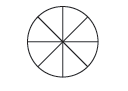CLASS ACTIVITYThe teacher• Gives each group of learners the sheet with fraction circles and fraction squares from the Printable Resources.• Discusses the following questions:−− Is one half bigger or smaller than one quarter? (Bigger.)−− Is one quarter bigger or smaller than one third? (Smaller.)−− What can you tell me about two quarters and a half? (They are the same size.)−− What can you tell me about one third and three quarters? (One third is smaller than three quarters/three quarters is bigger than one third.)• Helps learners to realise that even though the shapes differ, the fraction parts must always be found in thesame way – by sharing into equal sized parts. In other words a half is a half in relation to the whole.−− If the whole is a circle, half the circle is ‘half’.−− If the whole is a square, half the square is ‘half’.−− If the whole is four blocks, half of the blocks is two blocks.−− If the whole is 20 sweets, half of the sweets is ten sweets, and so on. • Asks questions about eighths, thirds, sixths and fifths as well. Talk about different wholes so that learners can generalise the concept of a whole and a fraction part of a whole. Pupils pay attention and participate STEP 3CLASS-WORK 1. Complete the fraction strips by filling in the fractions and then answer the questions below.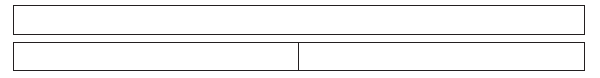2. Fill in bigger than/smaller than/equal to:a) One half is ______  three quartersb) Two quarters are _____  one halfc) Three quarters are ______  one thirdd) Three sixths are ______  four eighths3. How many eighths are equal to one whole? ___4. How many quarters are equal to three sixths? ____ Pupils attempt their class work STEP 4HOME-WORK 1. Draw a fraction table. Show the following: whole, halves, thirds, quarters, eighths.2. Give three examples where fractions are equal.(Various, e.g. two halves and one whole/two quarters and one half/six eighths and three quarters.) STEP 5SUMMARY The teacher summarizes by reminding the pupils how to recognize fractions in diagrammatic form She marks their class works, makes corrections where necessary and commends them positively

PERIOD 2 : Fractions-share and group things equally

 PRESENTATION TEACHER’S ACTIVITY PUPIL’S ACTIVITY STEP 1MENTAL MATHS The teacher begins the lesson with some mental calculationsCalculate1. 4 x 2 =2. 3 x 2 =3. 5 x 2 =4. 6 x 2 =5. 7 x 2 =6. 1 x 20 =7. 5 x 4 =8. 6 x 3 =9. 10 x 2 =10. 5 x 3 = Pupils respond and participate STEP 2CONCEPTDEVELOPMENT The teacher• Draws the following problems on the board.• Repeats the activity using different numbers.• Learners may use counters to assist them.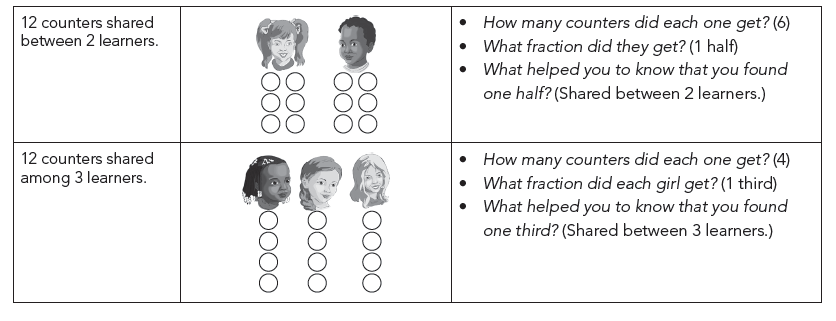CLASS ACTIVITYThe teacher* Writes the following problem on the board.Share 40 counters among 8 learners.Draw 8 circles then share the counters equally.• Asks the following questions:− How many counters will each one get? (5)− What fraction did they get? (1 eighth)− What is 1 eighth of 40? (5)− What is 2 eighths of 40? (10)− What is 3 eighths of 40? (15)− What is 4 eighths of 40? (20)− What is 5 eighths of 40? (25)− What is 6 eighths of 40? (30)− What is 7 eighths of 40? (35)− What is 8 eighths of 40? (40)− What is 1 half of 40? (20)− What is 1 quarter of 40? (10)− What is the whole of 40? (40) Pupils pay attention and participate STEP 3CLASS-WORK Calculate the following. Your answers must be written in number symbols.1. If 15 counters are shared among 3 learners:a) How many counters did each one get?b) What fraction did they get?c) What helped you to know that you found one third? 2. If 88 counters are shared among 8 learners:a) How many counters did each one get?b) What fraction did they get?c) What helped you to know that you found one eighth? 3. If 15 counters are shared among 5 learners:a) How many counters did each one get?b) What fraction did they get?c) What is 3 fifths of 15? 4. Share 30 counters into 6 groups.a) One sixth of 30 is ___.b) Two sixths of 30 is ___. 5. 12 is ___ of 24.6. 30 is ___ of 80. Pupils attempt their class work STEP 4HOME-WORK Complete the following. Use any way of writing fractions that you are comfortable with.1. 10 is ___ of 15. 2. 10 is ___ of 80. 3. Share 24 counters into 6 groups.a) One sixth of 24 is ___.b) Four sixths of 24 is ___. Pupils attempt their class work STEP 5SUMMARY The teacher summarizes by reminding the pupils how to share and group things equally She marks their class works, makes corrections where necessary and commends them positively

PERIOD 3: Fractions problems with unitary and non-unitary solutions

 PRESENTATION TEACHER’S ACTIVITY PUPIL’S ACTIVITY STEP 1MENTAL MATHS The teacher begins the lesson with some mental calculationsCalculate1. 10 x 10 =   2. 10 x 6 =     3. 10 x 8 =     4. 10 x 9 =     5. 10 x 1 =     6. 10 x 0 =  7. 10 x 2 =8. 10 x 4 =9. 10 x 3 =10. 10 x 5 = Pupils respond and participate STEP 2CONCEPTDEVELOPMENT The teacher• Does the following example practically and use drawings to illustrate it on the board.Three boys share 13 biscuits equally. How much will each boy get? 13 ÷ 3 = 4 rem 1So each boy gets 4 whole biscuits, and there is one biscuit left over.Can the friends share the one remaining biscuit? How?We cut the remaining biscuit into three equal parts so that each friend gets one piece.• Here is an illustration. You could show this insteps as you explain the sharing process.(The last biscuit has to be shared into thirds, so that each boy can get 1 third of the biscuit.)Now how much will each boy get? (4 and 1 third of a biscuit.)  CLASS ACTIVITYThe teacher• Does the next example practically and use drawings to illustrate on the board.Four friends share 7 bars of chocolate equally. How much will each friend get? 7 ÷ 4 = 1 rem 3So each friend gets 1 bar of chocolate, and there are 3 bars of chocolate left over.• Asks Can the friends share the three remaining bar of chocolate? How?We cut the remaining three bars of chocolate into four equal parts so that each friend gets one piece from each bar.(Each friend gets one whole bar of chocolate.)(The remaining 3 bars are divided into quarters and each friend getsone of the quarters from each bar – so each friend gets an extra 3quarters of a bar of chocolate.)Now how much will each friend get? (Each friend will get 1 and 3 quarters of a bar of chocolate.)• Shows learners how to write 1 and three quarters (1 and 3 quarters) on the board. ACTIVITY IIThe teacher• Writes the following two word problems on the board. Allow learners time to do the calculations on their whiteboards/scrap paper before they share their solutions with the whole class.−− Mum makes three skirts with 7 meters of material. All the skirts are the same size. How many metres of material does she use for one skirt? (2 and 1 third metres of material.)−− Eight teachers share 17 boxes of chalk. How many boxes of chalk will each teacher get? (2 and 1 eighth boxes of chalk.) Pupils pay attention and participate STEP 3CLASS-WORK 1. Find one quarter of 21 sweets. 2. Find 5/6 of 30 sweets.3. Share 9 chocolate bars among 4 friends so that they all get the same amount of chocolate and there is nothing left over. 4. Grandmother gives Kiki N12. Kiki wants to save â of the money. How much money should she save? 5. Four boys share 7 cakes. If they share them equally, how much cake do they each get? Pupils attempt their class work STEP 4HOME-WORK 1. Share 16 apples equally among 5 children so that they all get the same amount of apples and there isnothing left over. 2. Find three quarters of 60 sweets. 3. Eight boys share 7 cakes. If they share them equally, how much cake do they each get? Pupils attempt their class work STEP 5SUMMARY The teacher summarizes by reminding the pupils how to solve fraction problems with unitary and non-unitary solutions She marks their class works, makes corrections where necessary and commends them positively

PERIOD 4: Putting fractions together

 PRESENTATION TEACHER’S ACTIVITY PUPIL’S ACTIVITY STEP 1MENTAL MATHS The teacher begins the lesson with some mental calculationsCalculate1. 1 x 1 = 2. 4 x 5 = 3. 5 x 2 = 4. 3 x 1 = 5. 7 x 3 =  6. 3 x 4 =  7. 2 x 1 =8. 8 x 0 =9. 9 x 10 =10. 6 x 5 = Pupils respond and participate STEP 2CONCEPTDEVELOPMENT The teacher• Does the following questions practically and with drawings on the board.Mrs Gigi gives half an orange to each of her grandchildren. She had 14 grandchildren. How many oranges does she need?How many grandchildren? (14) How many oranges for each child? (one half) • Revises 1 orange can be cut into 2 halves.So to get 14 halves we need 7 oranges.• Asks: How many oranges does she need to make 14 halves? (She needs 7 oranges.) CLASS ACTIVITYThe teacher* writes the following problem on the boardI want to bake 10 apple pies. My recipe says that each pie takes a quarter of an apple.How many apples do I need?How many apple pies do I want to make? (10)How many apples in each? (1 quarter)How many quarters can I get from one apple? (4)How many apples do I need to get 10 quarters?(I need 3 apples, but I will not use all of the third apple. I will use 2 and a half apples.) • Draws 3 apples on the board.• Shows how to divide them into quarters.• Works together with the class to find out how many apples are needed to get 10 quarters.Ten quarters can be taken from 2 and a half apples.How many apples do I need? (I need 2 and a half apples.) ACTIVITY II• Learners draw and solve the following problems on their whiteboards/scrap paper.−− I drink one third of a glass of milk every day. How much milk will I drink in two weeks?(Remind learners that 1 week has 7 days. I will need 14 thirds = 4 and 2 thirds glasses of milk.)−− The driver uses one fifth of a tank of petrol each day. How many tanks of petrol will he use in 10 days?(2 tanks.) Pupils pay attention and participate STEP 3CLASS-WORK Draw pictures to help you to calculate.1. Mrs Seun gives a quarter orange to each of her grandchildren. She had 14 grandchildren.How many oranges does she need? 2. Mrs Lisa gives a third of an orange to each of her grandchildren. She had 14 grandchildren.How many oranges does she need? 3. Mrs Sheryl gives a fifth of an orange to each of her grandchildren. She had 14 grandchildren.How many oranges does she need? 4. Answer the following questions:a) Two quarters are the same as ___ .b) Four sixths are the same as ___ .c) Four eighths are the same as ___ .d) Six eighths are the same as ___ .e) One half is the same as ___ . Pupils attempt their class work STEP 4HOME-WORK 1. Label the fraction parts in the fraction wall:2. 2 quarters = ___ eighths3. 3 quarters = ___ eighths4. 1 half = ___ quarters5. 1 half = ___ eighths STEP 5SUMMARY The teacher summarizes by reminding the pupils how to put fractions together She marks their class works, makes corrections where necessary and commends them positively

PERIOD 5: Weekly Test/consolidations

TEACHER’S ACTIVITY: The teacher revises all the concepts treated from period 1-4 and gives the pupils follow through exercises, quiz and tests. She marks the exercises, makes corrections and commends the pupils positively.

PUPIL’S ACTIVITY: The pupils work on the worksheets and exercises given by the teacher individually

CONSOLIDATION